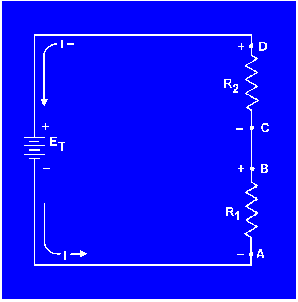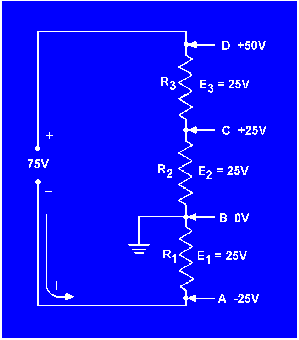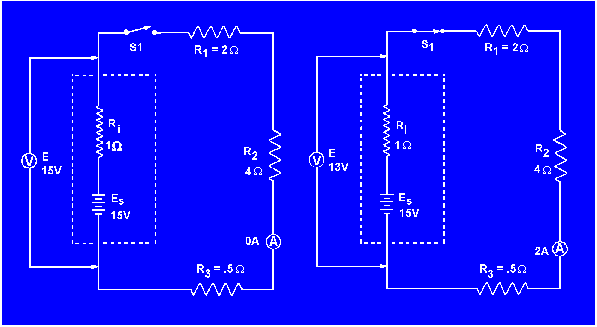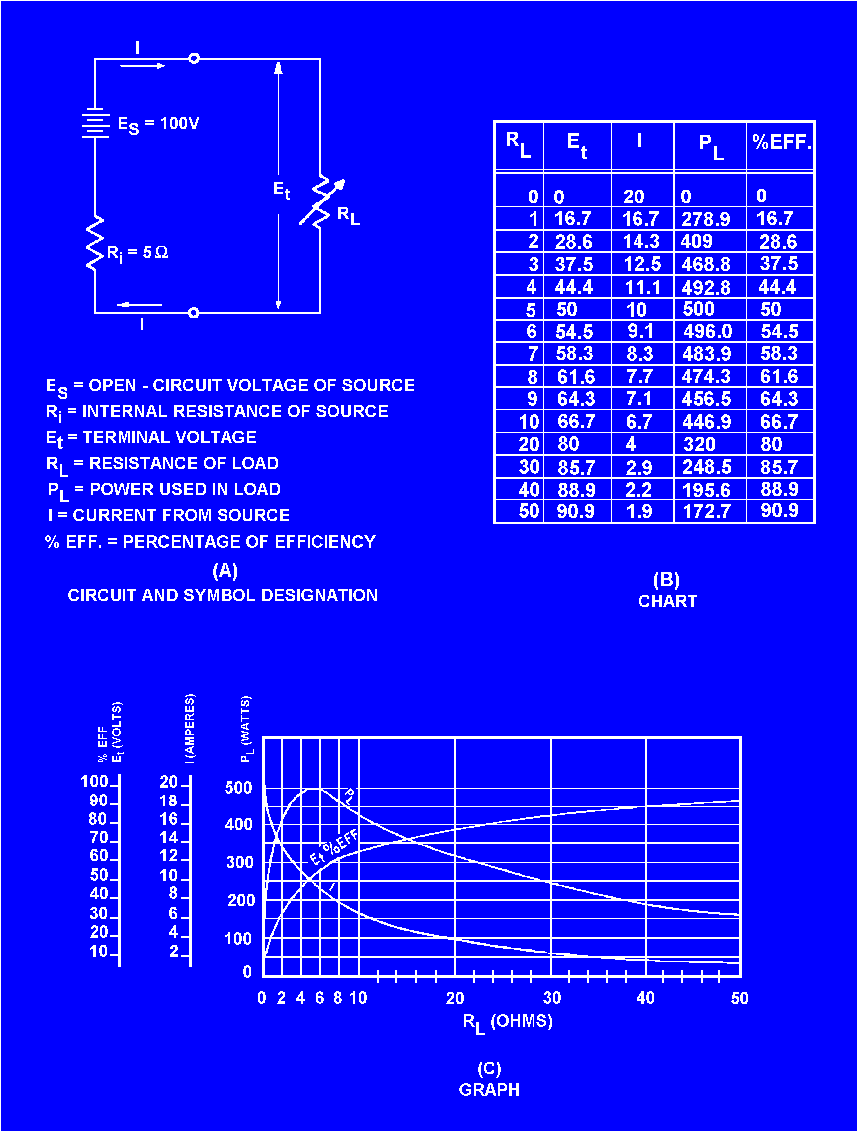Rules for series DC circuitsCustom SearchSummaryMatter, Energy, and ElectricityRules for parallel DC circuitsRULES FOR SERIES DC CIRCUITS:

The same current flows through each part of a series circuit.
The total resistance of a series circuit is equal to the sum of the individual resistances.
The total voltage across a series circuit is equal to the sum of the individual voltage drops.
The voltage drop across a resistor in a series circuit is proportional to the ohmic value of the resistor.
The total power in a series circuit is equal to the sum of the individual power used by each circuit component.

KIRCHHOFF'S VOLTAGE LAW states: The algebraic sum of the voltage drops in any closed path in a circuit and the electromotive forces in that path is equal to zero, or Ea + Eb + Ec +...En = 0.VOLTAGE POLARITIES must be used when applying Kirchhoff's voltage law. The point at which current enters a load (resistor) is considered negative with respect to the point at which current leaves the load.

SERIES AIDING VOLTAGES cause current to flow in the same direction; thus the voltages are added.SERIES OPPOSING VOLTAGES tend to force current to flow in opposite directions; thus the equivalent voltage is the difference between the opposing voltages.

A REFERENCE POINT is a chosen point in a circuit to which all other points are compared.AN OPEN CIRCUIT is one in which a break exists in the complete conducting pathway.

A SHORT CIRCUIT is an accidental path of low resistance which passes an abnormally high amount of current.

INTERNAL RESISTANCE causes a drop in the terminal voltage of a source as current flows through the source. The decrease in terminal voltage is caused by the voltage drop across the internal resistance. All sources of electromotive force have some form of internal resistance.HIGH EFFICIENCY in a circuit is achieved when the resistance of the load is high with respect to the resistance of the source.POWER TRANSFER in a circuit is highest when the resistance of the load equals the resistance of the source.

A PARALLEL CIRCUIT is a circuit having more than one current path connected to a common voltage source.Integrated Publishing, Inc. - A (SDVOSB) Service Disabled Veteran Owned Small Business